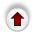# 4. Implementing a Tree in an Array

How can we represent an arbitrary binary tree in an array? In fact, there are numerous ways to do this, we'll just look at one.

Because an array's length is fixed at compile time, if we use an array to implement a tree we have to set a limit on the number of nodes we will permit in the tree. Our strategy is to fix the maximum height of the tree (H), and make the array big enough to hold any binary tree of this height (or less). We'll need an array of size (2**H)-1. Here is the biggest binary tree of depth 3:

`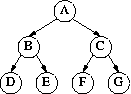`

If we picked H=3 as our limit, then every tree we might build will be a subtree of this one - this is the key insight behind our implementation.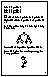What we do now is assign each of nodes to a specific position in the array. This could be done any way you like, but a particular easy and useful way is:

• root of the tree (A): array position 1
• root's left child (B): array position 2
• root's right child (C): array position 3
• ...
• left child of node in array position K: array position 2K
• right child of node in array position K: array position 2K+1
So D is in position 2*2 (4), E is in position 2*2+1 (5), F is in position 2*3 (6), G is in position 2*3+1 (7).

This figure shows the array position associated with each node:

`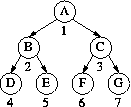`

This particular arrangement makes it easy to move from a node to its children, just double the node's index (and add 1 to go right). It also makes it easy to go from a node to its parent: the parent of node I has index (I div 2).

Using this strategy, a tree with N nodes does not necessarily occupy the first N positions in the array. For example, the tree:

`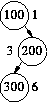`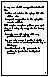Somehow we need to keep track of which array elements contain valid information. Two possibilities:

1. as usual, each node stores information saying which of its children exist

2. each array position stores information saying if it is a valid node.
However, if we restrict ourselves to complete trees, these problems go away. Because of the way we assigned nodes to positions, if there are N nodes in a complete tree, they will correspond to the first N positions of the array. So:
1. only need to keep track of N in order to know which array positions contain valid information.

2. if we add a new value, it must go in position N+1; if we delete a value, we must re-organize the tree so that the `gap' created by deleting is filled.

3. can traverse the tree by going through the array from first to Nth position.
`      for(i=0;i<N;i++) { process node in position i; }`
This gets us level-by-level traversal!

To see this `amazing' fact, look again at the earlier picture.

We have insisted that heaps be complete trees precisely so that they will have this very nice implementation in arrays. It is a useful exercise to work through the insert and delete operations for heaps in this array representation: the textbook gives code implementing INSERT and DELETE for this representation ((pp.279-80)).